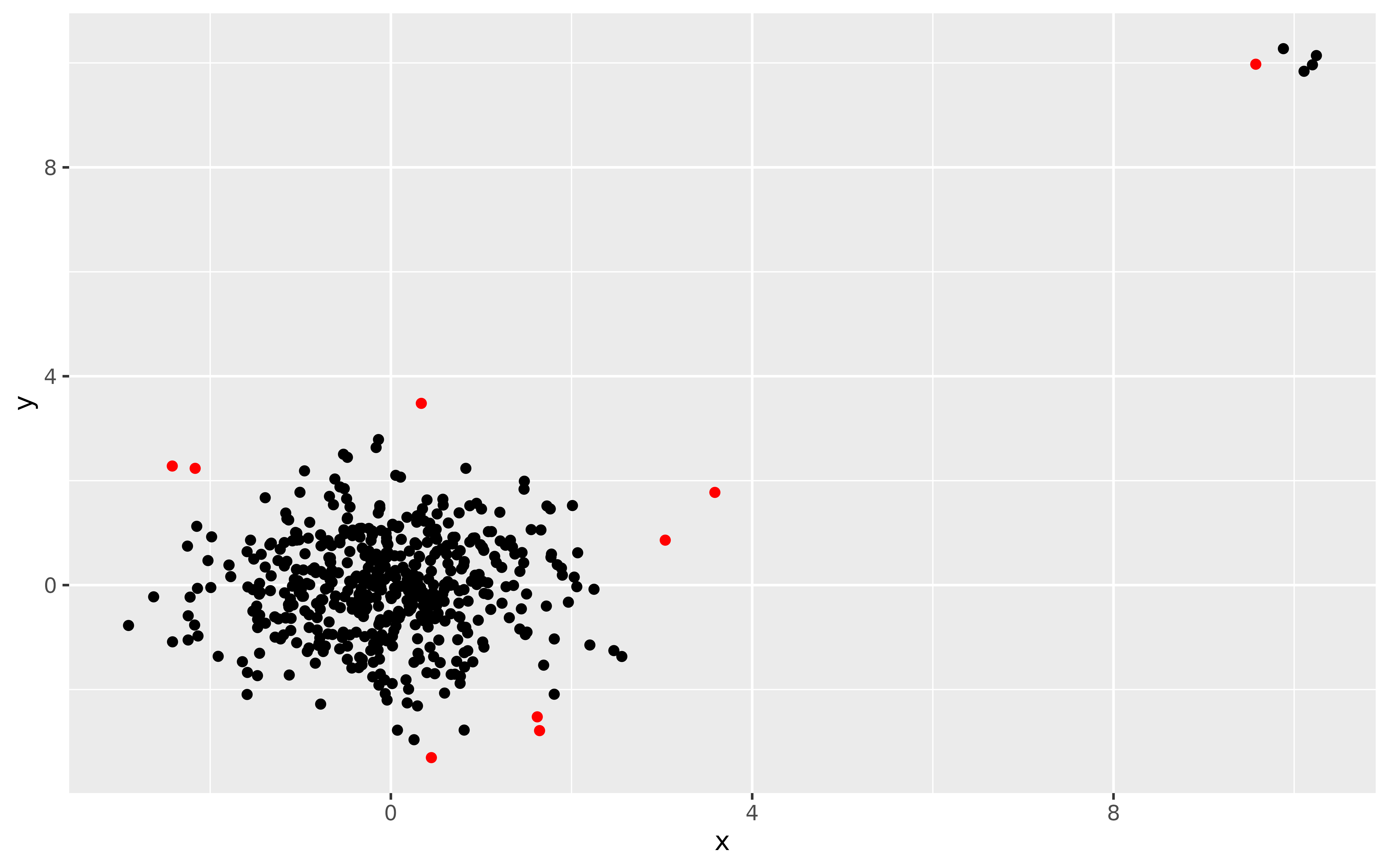This function identifies outliers using the algorithm lookout, an outlier detection method that uses leave-one-out kernel density estimates and generalized Pareto distributions to find outliers.

lookout(X, alpha = 0.05, unitize = TRUE, bw = NULL, gpd = NULL)

## Arguments

X The input data in a dataframe, matrix or tibble format. The level of significance. Default is 0.0.05. An option to normalize the data. Default is TRUE, which normalizes each column to [0,1]. Bandwidth parameter. Default is NULL as the bandwidth is found using Persistent Homology. Generalized Pareto distribution parameters. If NULL (the default), these are estimated from the data.

## Value

A list with the following components:

outliers

The set of outliers.

outlier_probability

The GPD probability of the data.

bandwidth

The bandwdith selected using persistent homology.

kde

The kernel density estimate values.

lookde

The leave-one-out kde values.

gpd

The fitted GPD parameters.

## Examples

X <- rbind(
data.frame(x = rnorm(500),
y = rnorm(500)),
data.frame(x = rnorm(5, mean = 10, sd = 0.2),
y = rnorm(5, mean = 10, sd = 0.2))
)
lo <- lookout(X)
lo
#> Leave-out-out KDE outliers using lookout algorithm
#>
#> Call: lookout(X = X)
#>
#>   Outliers Probability
#> 1      133  0.03770879
#> 2      501  0.02095786
#> 3      502  0.02006983
#> 4      503  0.01988786
#> 5      504  0.02048272
#> 6      505  0.02012449
#> autoplot(lo)